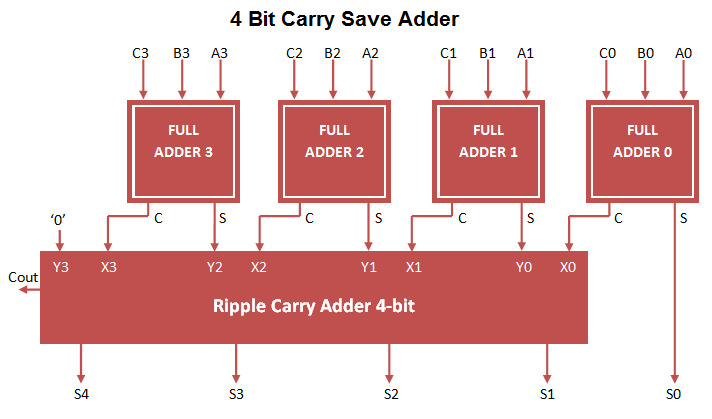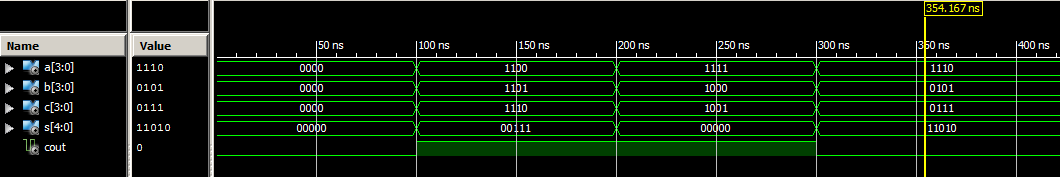# Carry Save Adder VHDL Code

Carry save adder used to perform 3 bit addition at once. Here 3 bit input (A, B, C) is processed and converted to 2 bit output (S, C) at first stage.  At first stage result carry is not propagated through  addition operation. In order to generate carry, implemented ripple carry adder on stage 2 for carry propagation. Carry Save adder VHDL Code can be constructed by port mapping full adder VHDL Code to 2 stage adder circuit.### Carry Save Adder VHDL Code

```
library IEEE;
use IEEE.STD_LOGIC_1164.ALL;

Port ( A : in STD_LOGIC_VECTOR (3 downto 0);
B : in STD_LOGIC_VECTOR (3 downto 0);
C : in STD_LOGIC_VECTOR (3 downto 0);
S : OUT STD_LOGIC_VECTOR (4 downto 0);
Cout : OUT STD_LOGIC);

Port ( A : in STD_LOGIC;
B : in STD_LOGIC;
Cin : in STD_LOGIC;
S : out STD_LOGIC;
Cout : out STD_LOGIC);
end component;

– Intermediate signal
signal X,Y: STD_LOGIC_VECTOR(3 downto 0);
signal C1,C2,C3: STD_LOGIC;

begin

end Behavioral;

```

### Testbench VHDL Code for Carry Save Adder

```
LIBRARY ieee;
USE ieee.std_logic_1164.ALL;

ENTITY Tb_carry_save IS
END Tb_carry_save;

ARCHITECTURE behavior OF Tb_carry_save IS

– Component Declaration for the Unit Under Test (UUT)

PORT(
A : IN std_logic_vector(3 downto 0);
B : IN std_logic_vector(3 downto 0);
C : IN std_logic_vector(3 downto 0);
S : OUT std_logic_vector(4 downto 0);
Cout : OUT std_logic
);
END COMPONENT;

--Inputs
signal A : std_logic_vector(3 downto 0) := (others => '0');
signal B : std_logic_vector(3 downto 0) := (others => '0');
signal C : std_logic_vector(3 downto 0) := (others => '0');

--Outputs
signal S : std_logic_vector(4 downto 0);
signal Cout : std_logic;

BEGIN

– Instantiate the Unit Under Test (UUT)
A => A,
B => B,
C => C,
S => S,
Cout => Cout
);

– Stimulus process
stim_proc: process
begin
– hold reset state for 100 ns.
wait for 100 ns;
A <= "1100";
B <= "1101";
C <= "1110";

wait for 100 ns;
A <= "1111";
B <= "1000";
C <= "1001";

wait for 100 ns;
A <= "1110";
B <= "0101";
C <= "0111";

wait;
end process;

END;

```

### Output Waveform for Carry Save adderCarry Save Adder performs Addition of 3 (A, B, C) 4-bit values and output 5 bit Sum and Cout. Example result (1100 + 1101 + 1110) = 100111 (MSB Bit ‘1’ Cout , Sum 5 bit ” 00111″).